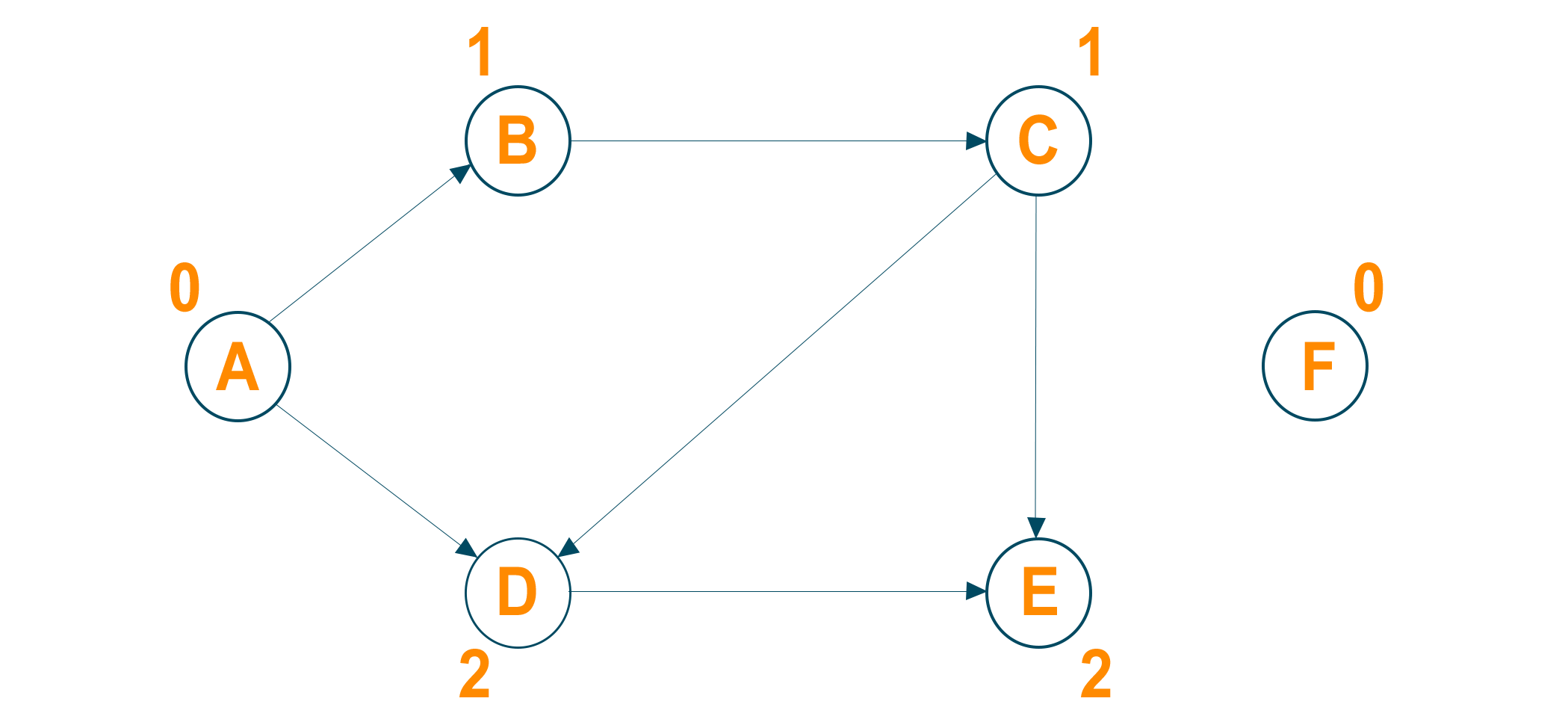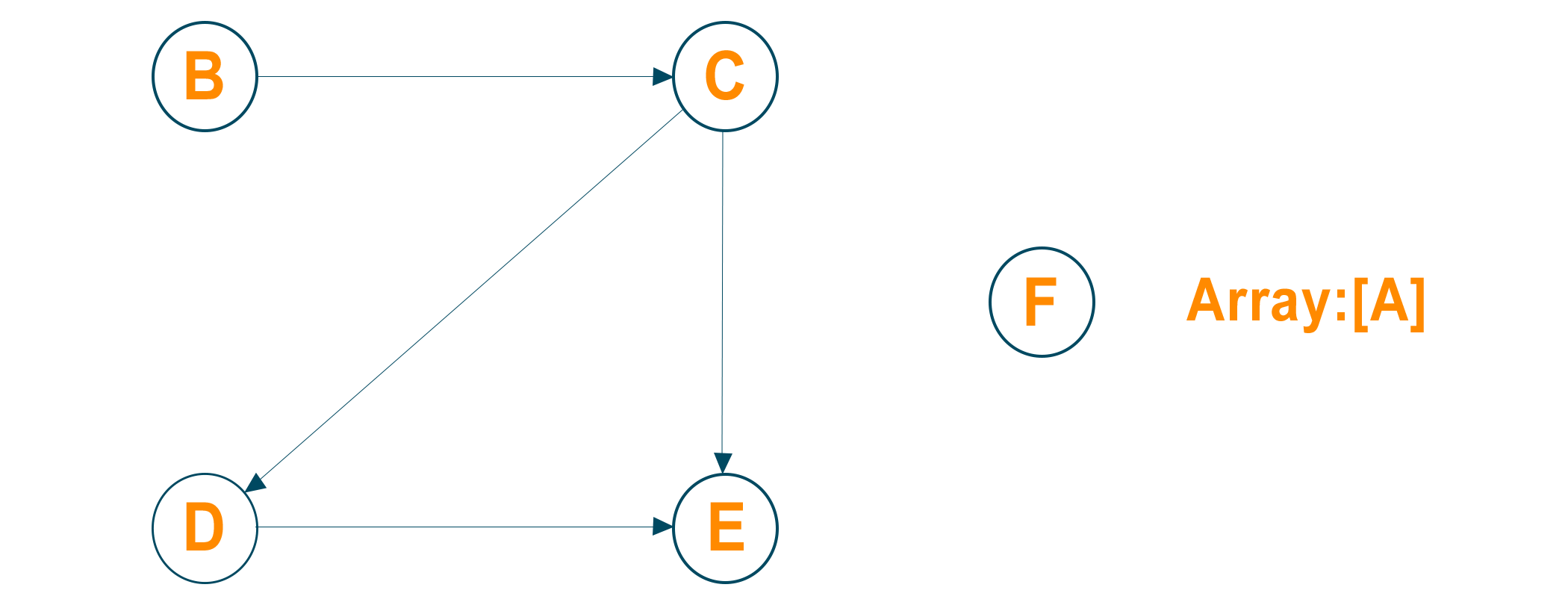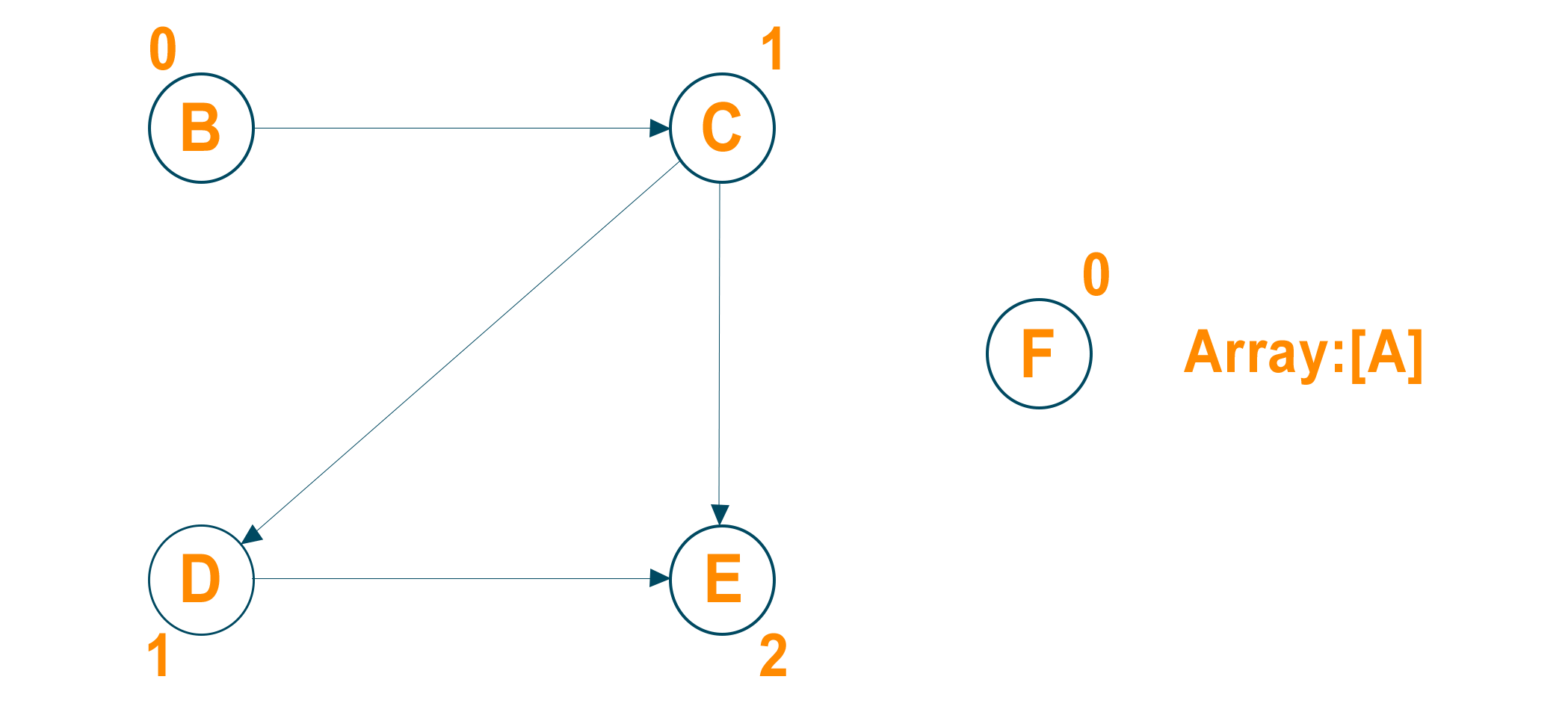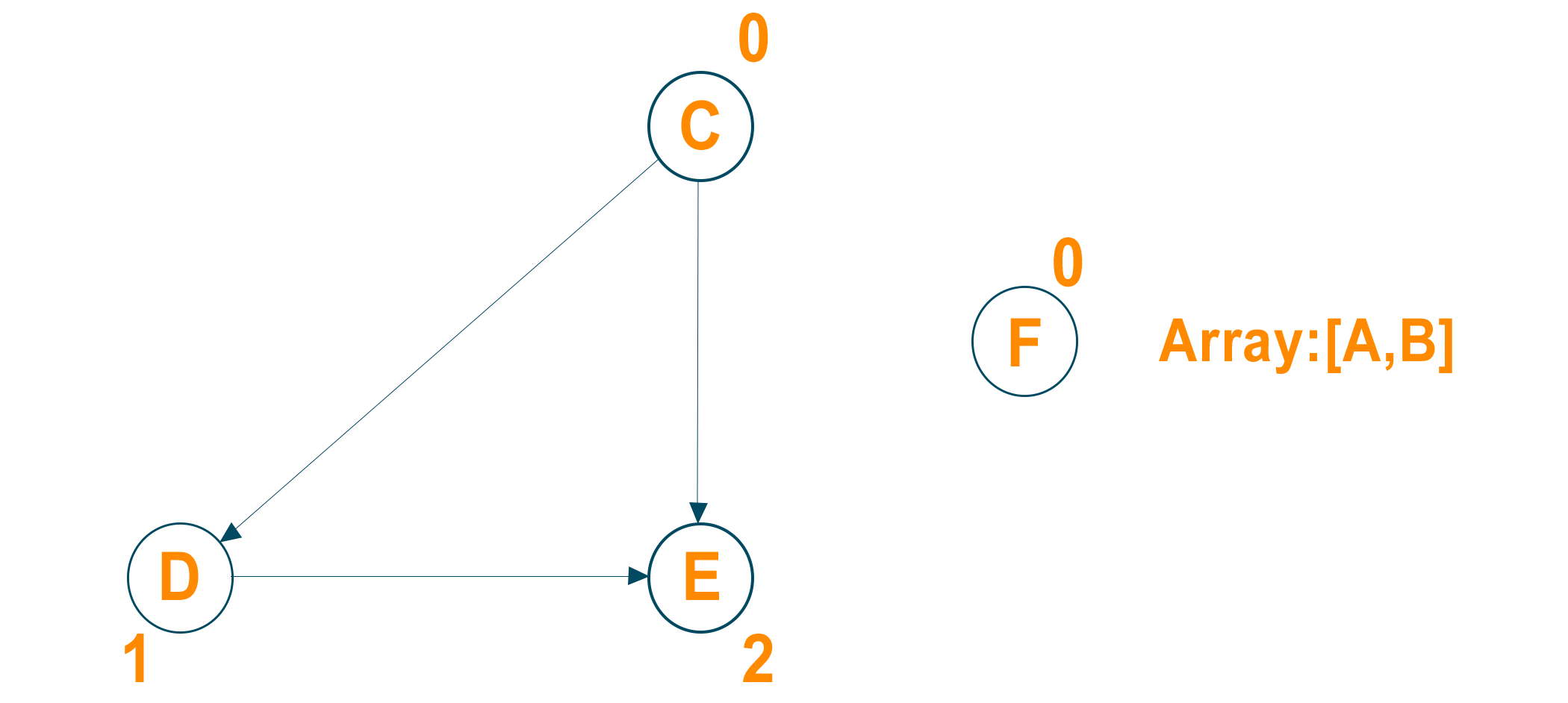# Topological Sort in Python for Directed Acyclic Graph (with code)

In this article, we will study what is topological sort and an example explaining how it works. We will also learn the implementation of topological sorting with Python program, the time complexity of the algorithm, and its applications. So, let’s get started!

## What is Topological Sort?

Topological sort is an algorithm that takes a directed acyclic graph and returns the sequence of nodes where every node will appear before other nodes that it points to.

Just to remind you, a directed acyclic graph (DAG) is a graph having directed edges from one node to another but does not contain any directed cycle. Remember topological sorting for graphs is not applicable if the graph is not a Directed Acyclic Graph (DAG). The ordering of the nodes in the array is called topological ordering.

Therefore we can say that a topological sort of the nodes of a directed acyclic graph is the operation of arranging the nodes in the order in such a way that if there exists an edge (i,j), i precedes j in the lists. A topological sort basically gives a sequence in which we should perform the job and helps us to check whether the graph consists of the cycle or not.

Every graph can have more than one topological sorting possible. It depends on the in-degree of the node in the graph. Also, the topological sorting of the graph starts with the node that has an in-degree of 0 i.e. a node with no incoming edges. Let us learn an example for a clear understanding.

### Topological Sorting Example

Consider the following directed acyclic graph with their in-degree mentioned.Identifying vertices that have no incoming edge. Here, nodes A and F have no incoming edges.

We will choose node A as the source node and deletes this node with all its outgoing edges and put it in the result array.Now, update the in-degree of the adjacent nodes of the source node after deleting the outgoing edges of node ANow again delete the node with in-degree 0 and its outgoing edges and insert it in the result array. Later update the in-degree of all its adjacent nodes.Now repeat the above steps to get the output below:In the second step, if we have chosen the source node as F then the topological sort of the graph will be F, A, B, C, D, E. Therefore, there is more than one topological sort possible for every directed acyclic graph.

### Topological Sort Algorithm

The algorithm of the topological sort goes like this:

1. Identify the node that has no in-degree(no incoming edges) and select that node as the source node of the graph
2. Delete the source node with zero in-degree and also delete all its outgoing edges from the graph. Insert the deleted vertex in the result array.
3. Update the in-degree of the adjacent nodes after deleting the outgoing edges
4. Repeat steps 1 to step 3 until the graph is empty

The resulting array at the end of the process is called the topological ordering of the directed acyclic graph. If due for some reason, there are some nodes left but they have the incoming edges, that means that the graph is not an acyclic graph and topological ordering does not exist.

## Python Program for Topological Sort

Here is the complete code to implement Topological Sorting in Python:

```from collections import defaultdict

class Graph:

def __init__(self,n):

self.graph = defaultdict(list)

self.N = n

self.graph[m].append(n)

def sortUtil(self,n,visited,stack):

visited[n] = True

for element in self.graph[n]:

if visited[element] == False:

self.sortUtil(element,visited,stack)

stack.insert(0,n)

def topologicalSort(self):

visited = [False]*self.N

stack =[]

for element in range(self.N):

if visited[element] == False:

self.sortUtil(element,visited,stack)

print(stack)

graph = Graph(5)

print("The Topological Sort Of The Graph Is:  ")

graph.topologicalSort()
```

Output:

```The Topological Sort Of The Graph Is:
[0, 1, 2, 3, 4]
```

### Time Complexity

The running time complexity of the topological sorting algorithm is O(M + N) where M is the number of edges in the graph and N is the number of nodes in the graph.

We have to determine the in-degree of each node in the graph which takes total O(M) time and then run the simple loop to place all the nodes in the result array by checking the in-degrees to be zero which takes total O(N) time for N number of nodes.

Therefore the total time complexity of the program will be O(M+N). The space complexity for the algorithm will be O(N) where N is the total number of nodes in the graph to allocate the nodes in the result array.

### Does topological sort use BFS or DFS?

Topological sorting is an algorithm that finds a linear ordering of nodes in a directed acyclic graph (DAG) such that node ‘u’ appears before node ‘v’ in the ordering for every directed edge (u, v).

In comparison to the BFS approach, the DFS approach is more obvious and clear. DFS traversal traverses all vertices recursively and then inserts the current vertex at the beginning of the result list. BFS traversal, on the other hand, discovers the in-degree of all vertices and then traverses the graph level by level, inserting vertices at the end of the result list.

The BFS approach, on the other hand, has several advantages over the DFS approach. The BFS technique, for example, is better suited for parallelization since it can be readily parallelized by conducting independent BFS traversals from each vertex with an in-degree of 0.

However, the implementation specifics and traversal order change between the two. To conduct topological sorting, both BFS (Breadth-First Search) and DFS (Depth-First Search) can be employed.

### What is the practical use of Topological Sort?

The ordering of vertices of a DAG (directed acyclic graph) with Topological Sorting can be used for a variety of practical applications some of those examples are:

• Task Scheduling: Topological sorting can also be used to plan project tasks. You may decide the order in which the jobs should be scheduled by modeling these relationships as a DAG and executing a topological sort.
• Course Scheduling: It can be used to build a course timetable at universities and colleges.
• Network routing: This sorting can be used to identify the order in which routers should be visited to route packets efficiently.
• Find Deadlock: Topological sort is useful to find the deadlock condition in an operating system and to find the dependency resolution.

## Conclusion

It is easy to determine the order of the compilation tasks to perform using the topological algorithm. With many different applications in real life, Topological Sorting in Python has its own importance while exploring and working with trees and graphs.

### FavTutor - 24x7 Live Coding Help from Expert Tutors!